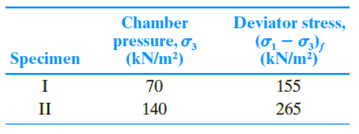Chapter 12, Problem 12.16PPrinciples of Geotechnical Enginee...

9th Edition
Braja M. Das + 1 other
ISBN: 9781305970939

Solutions

Chapter
SectionPrinciples of Geotechnical Enginee...

9th Edition
Braja M. Das + 1 other
ISBN: 9781305970939
Textbook Problem

The results of two consolidated-drained triaxial tests on a clayey sand are given here.Calculate the shear strength parameters of the soil.

To determine

Find the shear strength parameters of the soil.

Explanation

Given information:

Specimen 1.

The chamber pressure, σ3 70kN/m2.

The deviator stress is (σ1σ3)f is 155kN/m2

Specimen 2.

The chamber pressure, σ3 140kN/m2.

The deviator stress is (σ1σ3)f 265kN/m2

Calculation:

Show the expression to find the major effective principal stress for specimen 1 as shown below:

σ1=σ3tan2(45+ϕ2)+2c(45+ϕ2) (1)

Here, σ3 and σ1 minor and major effective principal stress, ϕ is angle of friction, and c is cohesion.

Substitute σ3+(Δσd)f for σ1 in Equation (1).

σ3+(Δσd)f=σ3tan2(45+ϕ2)+2c(45+ϕ2) (2)

Substitute 70kN/m2 for σ3 and 155kN/m2 for (Δσd)f in Equation (2).

70+155=70tan2(45+ϕ2)+2c(45+ϕ2)225=70tan2(45+ϕ2)+2c(45+ϕ2) (3)

Find the angle of friction using Equation (2) as follows:

Substitute 140kN/m2 for σ3 and 265kN/m2 for (Δσd)f in Equation (2)

Still sussing out bartleby?

Check out a sample textbook solution.

See a sample solution

The Solution to Your Study Problems

Bartleby provides explanations to thousands of textbook problems written by our experts, many with advanced degrees!

Get Started

What is a compounding interest rate?

Engineering Fundamentals: An Introduction to Engineering (MindTap Course List)

What kind of data and information can be found using a packet .sniffer?

Principles of Information Security (MindTap Course List)